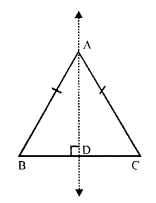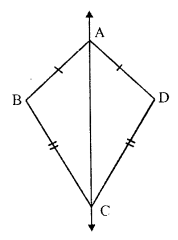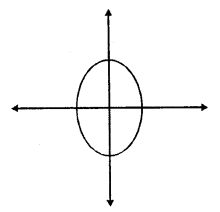## RS Aggarwal Class 7 Solutions Chapter 18 Reflection and Rotational Symmetry Ex 18A

These Solutions are part of RS Aggarwal Solutions Class 7. Here we have given RS Aggarwal Solutions Class 7 Chapter 18 Reflection and Rotational Symmetry Ex 18A.

Other Exercises

Tick (✓) the correct answer in each of Q.1 to Q.9
Question 1.
Solution:
(a) A scalene triangle has no line of symmetry

Question 2.
Solution:
(c) A rectangle has two lines of symmetry which are the lines joining the mid points of opposite sides.

Question 3.
Solution:
(d) A square has four lines of symmetry which are two diagonal and two the lines joining the mid points of opposite sides.

Question 4.
Solution:
(b) A rhombus has two lines of symmetry which are the diagonals.

Question 5.
Solution:
(d) A circle has an unlimited number of lines of symmetry as its lines of symmetry is its diameter which are infinite in number.

Question 6.
Solution:
(a) ∆ABC in which AB = AC, is an isosceles triangle and AD ⊥ BC.AD is the line of symmetry

Question 7.
Solution:
(a) ABCD is a kite in which AB = AD and BC = DCIts line of symmetry will be one diagonal AC.

Question 8.
Solution:
(c) The letter O of the English Alphabet has two lines of symmetry as shown here in the figure.Question 9.
Solution:
(a) The letter Z of the English Alphabet has no line of symmetry.

Question 10.
Solution:
The line/lines of symmetry have been drawn as given below :Question 11.
Solution:
(i) True
(ii) True
(iii) True : The bisectors of angles are its lines of symmetry.
(iv) False : A rhombus has two lines of symmetry which are its diagonals.
(v) True : The two diagonals and two perpendicular bisector of its opposite sides are the lines of symmetry.
(vi) True : The perpendicular bisectors of opposite sides are the two lines of symmetry of the rectangle.
(vii) True : Each of the English Alphabet H, I, O and X has two lines of symmetry.

Hope given RS Aggarwal Solutions Class 7 Chapter 18 Reflection and Rotational Symmetry Ex 18A are helpful to complete your math homework.

If you have any doubts, please comment below. Learn Insta try to provide online math tutoring for you.